# Pyramid vs Cube

Make a pyramid that looks like this: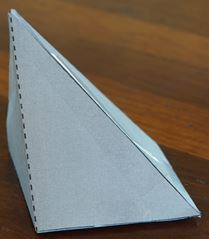• It has a square base
• Its apex (top point) is directly above one corner
• Its height (the distance from that corner to the apex) is the same as the base length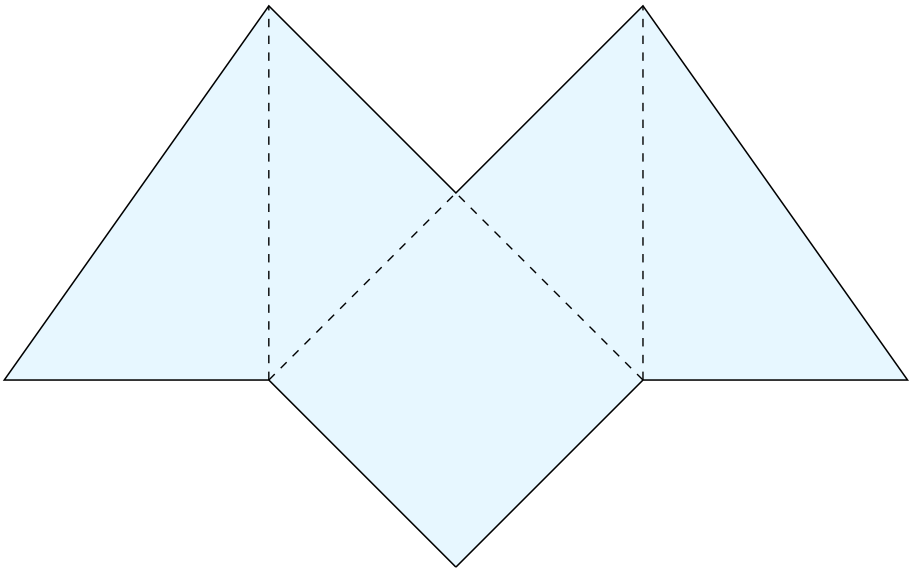Now make two more exactly the same, so we have three: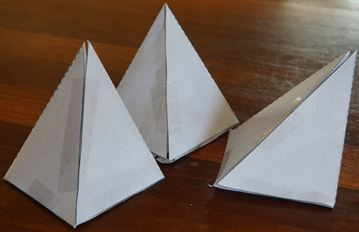Fit them together and we get a cube: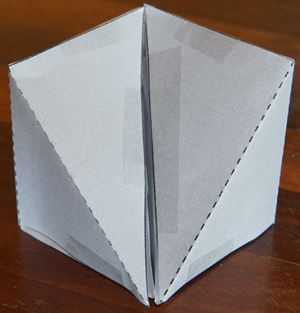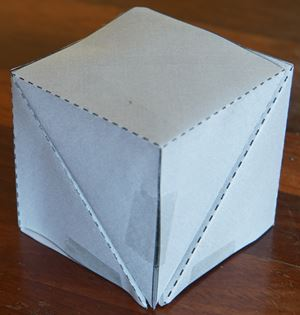So three of those pyramids exactly make one cube!

And so the volume of that pyramid is exactly one third of a cube.We can extend that idea to come up with the volume of a general pyramid:

Volume = 1 3  × [Base Area]× heightAnd, by thinking that a cone is just like a pyramid with a ridiculous number of sides, we can come up with the volume of a cone:

Volume = 1 3 π × r2 × h

Isn't mathematics wonderful!